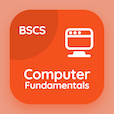Class 6 Online Courses

Grade 6 Math MCQ PDF - Chapters

# Factors and Multiples MCQ Quiz Online p. 8

Solve Factors and multiples quiz questions and answers PDF, factors and multiples trivia questions with answers to learn grade 6 math worksheet 8 for courses online. Prime and Composite Numbers MCQs, factors and multiples quiz questions and answers for online learning. "Factors and Multiples Quiz" PDF eBook: prime and composite numbers, highest common factor, prime factorization, index notation test prep for distance learning.

"The largest prime number less than 20 is" Multiple Choice Questions (MCQ) on factors and multiples with choices 15, 16, 19, and 18 for online learning. Study prime and composite numbers quiz questions for school certificate programs for online study.

## MCQs on Factors and Multiples Quiz

MCQ: The largest prime number less than 20 is

16
15
19
18

MCQ: The HCF of 450 and 540 is

30
24
20
16

MCQ: The numbers that have only two factors and are different from each other are called

odd numbers
prime numbers
composite numbers
even numbers

MCQ: The prime factors of 40 are

2 x 2 x 3 x 5 x 5
2 x 3 x 5 x 5
2 x 2 x 2 x 5
2 x 3 x 5

MCQ: The prime factors of 98 in the form of index notation are

2 x 5 x 7²
2 x 5²
2 x 7²
2 x 7³